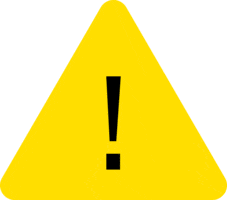# Math Worksheets Land

Math Worksheets For All Ages

# Math Worksheets Land

Math Worksheets For All Ages

Home > Math Topics > Trigonometry >

# Reference Angles Worksheets

When you first see this topic, you feel as that the concept of reference is something that will be provided to you, but a reference angle is something that you will need to determine on your own. It is defined as the shallowest potential angle that is created by the ending side of a predetermined angle with the x-axis. It is never an obtuse angle. Once you are able to define the measure of this angle, it kicks everything else off and you should not have much issue determining the value of the other two. Through this series of worksheets and lessons, students learn how to use and manipulate known values of angles to determine other values in the same system.

### Aligned Standard: HSF-TF.C.8

• Answer Keys - These are for all the unlocked materials above.
• Homework Worksheet 1 - Example Question: The angles of a triangle are in the ratio of 4:5:6. Find the measure of all the interior angles of the triangle.
• Homework Worksheet 2 - Example Question: In triangle SDF, ∠S = (x + 32), ∠D = (x + 14) and ∠F = 54°. Find the measures of ∠S and ∠D.
• Practice Worksheet 1 - Example Question: The vertex angle of an isosceles triangle measures 40°. Find the measure of both base angles.
• Quiz 1 - Example Question: Using Heron's formula, find the area of the triangle, to the nearest tenth.
• Quiz 2 - Example Question: Find the measure of ∠3, if ∠4 = 78° and ∠2 = 32° in the given diagram.

### What are Reference Angles in Trigonometry?When the terminal side of the angle makes the smallest angle with the x-axis, that is what we call reference angles in trigonometry. The value of the angle is always positive, regardless of the quadrant in which it lies and is always less than 90˚. It can be equal to 90˚, but it can never be greater, even for large angles. It is useful when using trigonometric functions. When angles have the same reference angles, they give the same trigonometric function values. It eliminates the need to memorize trigonometric function values for angles less than or equal to 90˚.

It is very easy to find reference angles. When dealing with large angles, you need to subtract 360˚ from it until you get an angle between 0˚ and 360˚. For negative angles, you add 360˚, until you get an angle within the range from 0˚ to 360˚. You can then sketch the angle to determine which quadrant the reference angle lies in.

If the angle lies in the 1st quadrant, it is the reference angle. If it lies in the second quadrant, you subtract the angle from 180˚. For the third quadrant, you subtract 180˚ from the angle, and for the fourth quadrant, you subtract 360˚ from the angle.

When you make movements around reference angles, it is often helpful to understand the terminology that is attributed to them. A revolution is a complete movement around a fixed object, so that wherever you start from you end up at. If you remember the game duck-duck-goose, it is going around the circle and stopping where you got up from. In two-dimensional geometry this is often considered a 360˚ angle movement.

Unlock all the answers, worksheets, homework, tests and more!
Save Tons of Time! Make My Life Easier Now

## Thanks and Don't Forget To Tell Your Friends!

I would appreciate everyone letting me know if you find any errors. I'm getting a little older these days and my eyes are going. Please contact me, to let me know. I'll fix it ASAP.